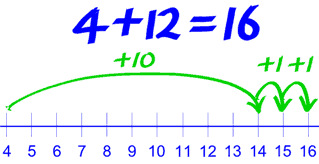## Monday, 20 November 2017

In Maths lessons over the last week 1/2 F students haves been learning to use a variety of strategies to solve addition and subtraction problems involving one- and two-digit numbers.

We have used the Jump Strategy:

Jumping by parts of a number to solve a calculation.

For example: 4 + 12 = 4 + 10 + 1 + 1Students have learnt to work backwards through some problems to convert them from additions to subtractions e.g. 8 + 10 =  … + 10 = 18

We have had lots of fun learning about these strategies and practiced using them by playing games and solving riddles.

Some the riddles solved were quite funny!
What do you call a snowman's tantrum? A meltdown
What do you call a cat on a beach? Sandy Claws